# Maps¶

Like for other plots, there are different approaches in R to make maps. You can use “base plot” in many cases. Alternatively use levelplot, either via the `spplot` function (implemented in `sp` and `raster`) or via the `rasterVis` package.

Here are some brief examples about making maps. You can also look elsewhere on the Internet, like here, or this for spplot and rasterVis.

## Vector data¶

### Base plots¶

```library(raster)
p <- shapefile(system.file("external/lux.shp", package="raster"))
plot(p)
```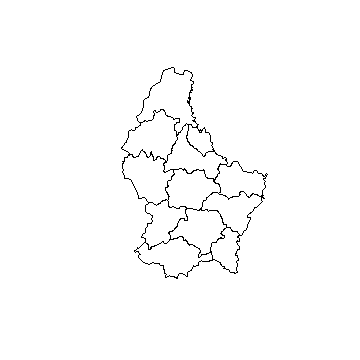```n <- length(p)
plot(p, col=rainbow(n))
```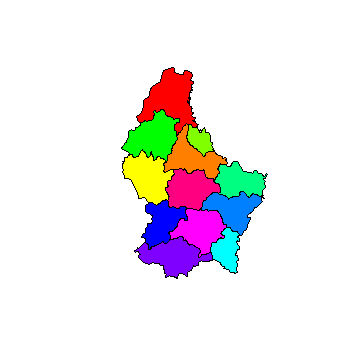One colour per region (NAME_1)

```u <- unique(p\$NAME_1)
u
##  "Diekirch"     "Grevenmacher" "Luxembourg"
m <- match(p\$NAME_1, u)
plot(p, col=rainbow(n)[m])
text(p, 'NAME_2', cex=.75, halo=TRUE)
```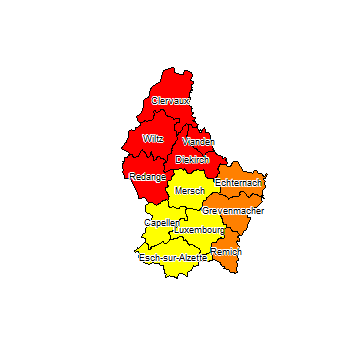### spplot¶

```spplot(p, 'AREA')
```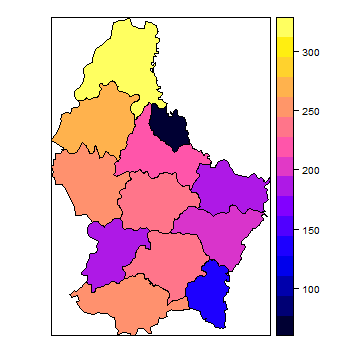## Raster¶

Example data

```library(raster)
b <- brick(system.file("external/rlogo.grd", package="raster"))
r <- raster(p, res=0.01 )
values(r) <- 1:ncell(r)
```

Several generic functions have been implemented for Raster* objects to create maps and other plot types. Use ‘plot’ to create a map of a Raster* object. When plot is used with a `RasterLayer`, it calls the function ‘rasterImage’ (but, by default, adds a legend; using code from `fields::image.plot`). It is also possible to directly call `image`. You can zoom in using ‘zoom’ and clicking on the map twice (to indicate where to zoom to). With `click` it is possible to interactively query a Raster* object by clicking once or several times on a map plot.

After plotting a `RasterLayer` you can add vector type spatial data (points, lines, polygons). You can do this with functions points, lines, polygons if you are using the basic R data structures or plot(object, add=TRUE) if you are using Spatial* objects as defined in the sp package. When plot is used with a multi-layer Raster* object, all layers are plotted (up to 16), unless the layers desired are indicated with an additional argument.

```plot(r)
```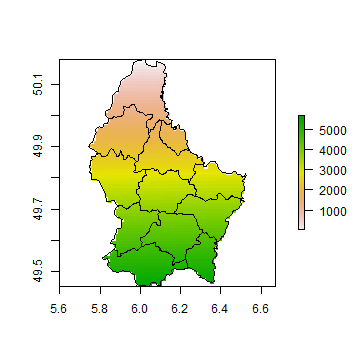`image` does not provide a legend and that can be advantageous in some cases.

```image(r)
```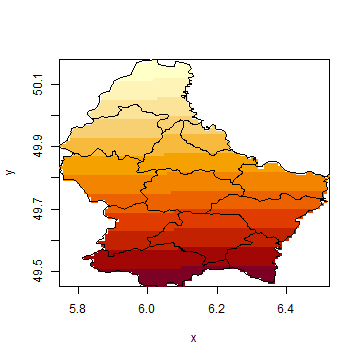Multi-layer Raster objects can be plotted as individual layers

```plot(b)
```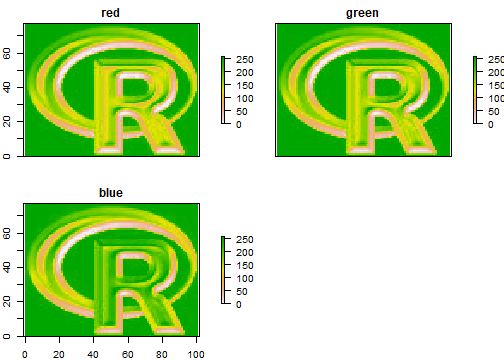They can also be combined into a single image, by assigning individual layers to one of the three color channels (red, green and blue):

```plotRGB(b, r=1, g=2, b=3)
```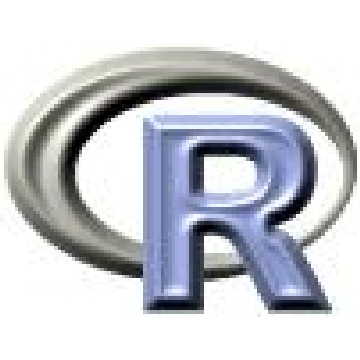You can also plot `Raster*` objects with spplot.

```bounds <- list("sp.polygons", p)
spplot(r, sp.layout=bounds)
```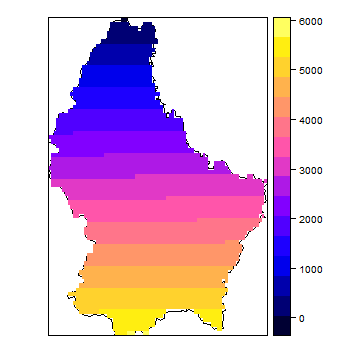```spplot(b, layout=c(3,1))
```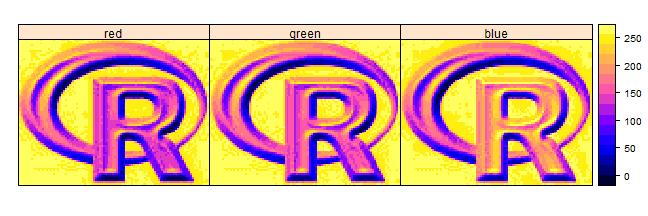The `rasterVis` package has several other `lattice` based plotting functions for `Raster*` objects. The rasterVis package also facilitates creating a map from a `RasterLayer` with the `ggplot2` package.

You can also use a number of other plotting functions with `raster` objects, including `hist`, `persp`, `contour`}, and `density`. See the help files for more info.

coming soon….